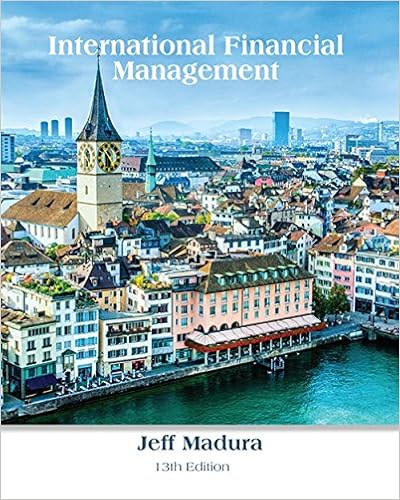# Assume that in recent months most currencies of

• Test Prep
• ChancellorBraverySandpiper10149
• 10
• 100% (34) 34 out of 34 people found this document helpful

This preview shows page 5 - 8 out of 10 pages.

##### We have textbook solutions for you!
The document you are viewing contains questions related to this textbook.The document you are viewing contains questions related to this textbook.
Chapter 21 / Exercise 14
International Financial ManagementExpert Verified
19. Assume that in recent months, most currencies of industrialized countries depreciated substantially against the dollar. Assume that their interest rates were similar to the U.S. interest rate. If non-U.S. firms invested in U.S. Treasury securities during this period, their effective yield would have been:A) negative.B) zero.C)positive, but less than the interest rate of their respective countries.D)more than the interest rate of their respective countries.ANSWER:
D 20. According to _______, the effective yield earned by U.S. investors will be the same as the effective yield earned by non-U.S. investors in any given period.
D 21. Assume Costner Corporation, a U.S.-based MNC, invests 2,500,000 Zambian kwacha (ZMK) for a one-year period at a nominal interest rate of 9%. At the time the loan is extended, the spot rate of the kwacha is \$.00060. If the spot rate of the kwacha in one year is \$.00056, the dollar amount initially invested in Zambia is \$__________, and \$___________ are paid out after one year.
A
##### We have textbook solutions for you!
The document you are viewing contains questions related to this textbook.The document you are viewing contains questions related to this textbook.
Chapter 21 / Exercise 14
International Financial ManagementExpert Verified
90International Financial Management22.Bullock Corporation invests 1,500,000 South African rand at a nominal interest rate of 10%. At the time the investment is made, the spot rate of the rand is \$.205. If the spot rate of the rand at maturity of the investment is \$.203, what is the effective yield of investing in rand?
B
23.Assume that interest rate parity holds. The U.S. one-year interest rate is 10% and the Australian one-year interest rate is 8%. What will the approximate effective yield be for an Australian citizen of a one-year deposit denominated in U.S. dollars? Assume the deposit is covered by a forward sale of dollars.A) 10%.B) 8%.C) 2%.D)cannot answer without more informationANSWER: SOLUTION: If interest rate parity holds, the Australian citizen will be able to earn approximately his or her domestic interest rate.
B
24.Assume that you forecast the value of the euro as follows for the next year:Percentage ChangeProbability of Occurrence-2%30%3%40%5%30%If the interest rate on the euro is 12%, the expected effective yield from a euro-denominated deposit is:
D
Chapter 21: International Cash Management91
The following information refers to questions 25 and 26. To benefit from the low correlation between the Trinidad dollar and the Japanese yen (¥), Sciorra Corporation decides to invest 50% of total funds invested in Trinidad dollars and the remainder in yen. The domestic yield on a one-year deposit is 8%. The Trinidad one-year interest rate is 10% and the Japanese one-year interest rate is 7%. Sciorra has determined the following possible percentage changes in the two individual currencies as follows:CurrencyPercentage Change
•••